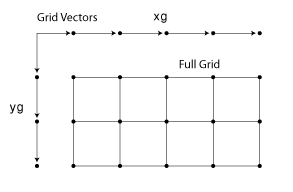Main Content

# ndgrid

Rectangular grid in N-D space

## Syntax

``````[X1,X2,...,Xn] = ndgrid(x1,x2,...,xn)``````
``````[X1,X2,...,Xn] = ndgrid(xg)``````

## Description

example

``````[X1,X2,...,Xn] = ndgrid(x1,x2,...,xn)``` replicates the grid vectors `x1,x2,...,xn` to produce an `n`-dimensional full grid.```

example

``````[X1,X2,...,Xn] = ndgrid(xg)``` specifies a single grid vector `xg` to use for all dimensions. The number of output arguments you specify determines the dimensionality `n` of the output.```

## Examples

collapse all

Create a 2-D grid from the vectors `[1 3 5 7 9 11 13 15 17 19]` and `[2 4 6 8 10 12]`.

`[X,Y] = ndgrid(1:2:19,2:2:12)`
```X = 10×6 1 1 1 1 1 1 3 3 3 3 3 3 5 5 5 5 5 5 7 7 7 7 7 7 9 9 9 9 9 9 11 11 11 11 11 11 13 13 13 13 13 13 15 15 15 15 15 15 17 17 17 17 17 17 19 19 19 19 19 19 ```
```Y = 10×6 2 4 6 8 10 12 2 4 6 8 10 12 2 4 6 8 10 12 2 4 6 8 10 12 2 4 6 8 10 12 2 4 6 8 10 12 2 4 6 8 10 12 2 4 6 8 10 12 2 4 6 8 10 12 2 4 6 8 10 12 ```

Evaluate and plot the function

`${x}_{1}{e}^{-{x}_{1}^{2}-{x}_{2}^{2}}$`

over the gridded domain

$-2<{x}_{1}<2$ and $-2<{x}_{2}<2$.

Create a grid of values for the domain.

`[X1,X2] = ndgrid(-2:.2:2);`

Evaluate the function over the domain.

`Z = X1 .* exp(-X1.^2 - X2.^2);`

Generate a mesh plot of the function.

`mesh(X1,X2,Z)`In R2016b and later releases, this task does not require the use of `ndgrid`. Instead, you can construct the grid using implicit expansion with these commands:

`x = -2:.2:2;`

`Z1 = x.' .* exp(-(x.').^2 - x.^2);`

Create a 2-D grid and calculate some function values on the grid. Interpolate between the assigned values to refine the grid.

Create a coarse grid for $\left(x,y\right)$ in the range $\left[-5,5\right]$.

`[X,Y] = ndgrid(-5:0.5:5);`

Calculate some function values on the grid and plot the function.

```f = sin(X.^2) * cos(Y.^2); surf(X,Y,f)```Interpolate between the points using a more refined grid and plot the result.

```[X1,Y1] = ndgrid(-5:0.125:5); F = interpn(X,Y,f,X1,Y1,'spline');```
`surf(X1,Y1,F)`## Input Arguments

collapse all

Grid vectors, specified as vectors containing grid coordinates for each dimension. The grid vectors implicitly define the grid. For example, in 2-D:Data Types: `single` | `double` | `int8` | `int16` | `int32` | `int64` | `uint8` | `uint16` | `uint32` | `uint64`
Complex Number Support: Yes

Grid vector for all dimensions, specified as a vector containing grid coordinates. `ndgrid` uses `xg` as the grid vector for each dimension.

Data Types: `single` | `double` | `int8` | `int16` | `int32` | `int64` | `uint8` | `uint16` | `uint32` | `uint64`
Complex Number Support: Yes

## Output Arguments

collapse all

Full grid representation, returned as separate arrays. For each output array `Xi`, the `i`th dimension contains copies of the grid vector `xi`.

## More About

collapse all

### Convert Between `meshgrid` and `ndgrid` Formats

`meshgrid` and `ndgrid` create grids using different output formats. Specifically, the first two dimensions of a grid created using one of these functions are swapped when compared to the other grid format. Some MATLAB® functions use grids in `meshgrid` format, while others use `ndgrid` format, so it is common to convert grids between the two formats.

You can convert between these grid formats using `pagetranspose` (as of R2020b) or `permute` to swap the first two dimensions of the grid arrays. For example, create a 3-D grid with `meshgrid`.

`[X,Y,Z] = meshgrid(1:4,1:3,1:2);`

Now transpose the first two dimensions of each grid array to convert the grid to `ndgrid` format, and compare the results against the outputs from `ndgrid`.

```Xt = pagetranspose(X); Yt = pagetranspose(Y); Zt = pagetranspose(Z); [Xn,Yn,Zn] = ndgrid(1:4,1:3,1:2); isequal(Xt,Xn) & isequal(Yt,Yn) & isequal(Zt,Zn)```
```ans = logical 1```

Using `pagetranspose` is equivalent to permuting the first two dimensions while leaving other dimensions the same. You can also perform this operation using `permute(X,[2 1 3:ndims(X)])`.

## See Also

### Topics

Introduced before R2006a

Download ebook